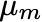# Moonfall – The Movie

Moonfall is a movie coming out in 2022. It creates a scenario where the Moon’s orbit is changed and set on a collision course with the Earth. It is fun to work out the orbital mechanics.

Let us assume that the Moon is in a circular orbit around the Earth. It is actually more influenced by the Sun than the Earth, but the circular orbit approximation is sufficient for our purposes. A mysterious force changes the orbit from circular to elliptical so that at closest approach it hits the Earth. The transfer orbit has an eccentricity of 0.9673 and a semi-major axis of 195000 km. The new orbital period is 9.9 days so it will hit the Earth in 5 days!

What kind of force is needed? The required velocity change is 0.83 km/s so a force of 6 x 1016 N applied over 10 seconds is required. To get an idea of how large that force really is, the Space Launch System (SLS) Block 2 vehicle produces about 10 million pounds of thrust , which is approximately 50 x 106 N (50 MN). Hence it would take 1.2 billion SLS rockets firing for 10 seconds to perform such a re-direction of the Moon! An image of the SLS is shown below (image from ).

As the Moon approaches the Earth it is going to raise the tides. A simple formula (really only valid when the Moon is far from the Earth) is

whereis the gravitational constant for the moon,is the gravitational constant for the Earth, r is the distance between the Earth and Moon and a is the radius of the Earth. The distance during the approach and the wave height are shown in the following plot.

By around 3 days the tides started getting really big! We’d expect the Moon’s gravitational force also to pull on the solid part of the Earth’s surface, causing all sorts of trouble.

References:

 https://www.nasa.gov/sites/default/files/atoms/files/0080_sls_fact_sheet_10092018.pdf